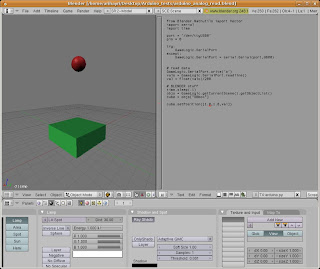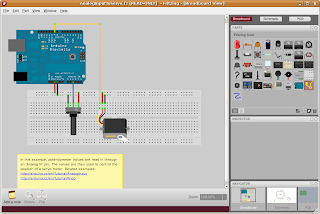## maanantai 20. huhtikuuta 2009

### /dev/Arduino

I noticed that if I unplugged Arduino from my Ubuntu-box and then plugged it back, the /dev name for Arduino changed from /dev/ttyUSB0 to /dev/ttyUSB1. Fortunately this was easy to fix. The problem was solved by adding a file 97-arduino.rules in /etc/udev/rules.d/ and adding the following rule (all in one line):
`kernel=="ttyUSB*", SYSFS{idVendor}=="0403", SYSFS{idProduct}=="6001", SYMLINK="Arduino"`

So now I can always trust that Arduino is in /dev/Arduino. If you have two Arduinos,you can use serial numbers in order to recognize them (see link below).

Original source:
http://www.arduino.cc/playground/Linux/Udev

## perjantai 17. huhtikuuta 2009

### Bouncing virtual ball with Arduino (and Blender)

UPDATED 20.4.2009I'm quite new on this Arduino stuff and I may have misunderstood something. But anyway, here are some observations of using Arduino and Blender's game engine.

## It's easy!

It is really easy to get values from Arduino to Blender. Here is a simple setup for reading photo-resistor's values from Blender:
```from Blender.Mathutils import Vector import serial
port = '/dev/ttyUSB0'
pin = 0
try:
GameLogic.SerialPort
except:
GameLogic.SerialPort = serial.Serial(port,9600)

# read data
valo = GameLogic.SerialPort.readline()
val = float(valo)/200

# BLENDER stuff

objs = GameLogic.getCurrentScene().getObjectList()
cube = objs["OBbox"]
cube.setPosition([1.0,1.0,val])```

Here I'm reading photoresistor values and then tell Blender's game-engine to change "box" object's position according that value. The arduino code looks like this:

```/* Arduino reading photo resistor to serial * * */
int analogPin=0;
int analogVal=0;
void setup() { Serial.begin(9600); }
void loop() {
// get the value from photoresistor
analogVal = analogRead(analogPin);
Serial.println(analogVal);
//delay(100);
}```

## Not so fast!

There is a one catch when reading data from Arduino to Blender. And that's the fact that python scripts are executed on every frame and that mean that you'll read data from serial port approx. 60 times per second.

However, Arduino is sending data much faster. As a result, you'll see a horrible delay in Blender. That's because the data arduino sent is buffered and you are actually reading from buffer. So you'll get "historical" values from serial port, not the current values. And for interactive application that is not wanted.

There are couple of ways to fix this:

The simplest way is to program Arduino to send data more rarely. You could use delay function if there is nothing else that Arduino should do (delay halts everything in Arduino).

## Request/response model

Better and more predictable way is to tell Arduino when we want to get a value. So when we want a value, we send a signal to arduino to send data and then we read the value. This way we'll always get the current values no matter of how high or low our frame rates are.

(I *think* that it would be also possible to run a separate python thread for Arduino input and just read values from that thread)

```port = '/dev/Arduino'
pin = 0

try:
GameLogic.SerialPort
except:
try:
GameLogic.SerialPort = serial.Serial(port,9600,timeout=1)
print 'Port ' + port + 'found!'

#ok, we have a port. Let's test if we can write to it
try:
GameLogic.SerialPort.write('x')
GameLogic.serialRead = False
GameLogic.serialOk = True

except:
GameLogic.serialOk = False
print 'could not write to port ' + port + '!'

except:
GameLogic.serialOk = False
print 'no port ' + port + ' found!'

# do stuff if serial port is OK
if GameLogic.serialOk:
if GameLogic.serialRead:
valo = GameLogic.SerialPort.readline()
val = float(valo)/100
GameLogic.serialRead = False
else:
GameLogic.SerialPort.write('a')
GameLogic.serialRead = True

# BLENDER stuff
if GameLogic.serialRead == False:
objs = GameLogic.getCurrentScene().getObjectList()
cube = objs["OBbox"]
cube.setPosition([1.0,1.0,val])```

When Blender is ready to receive a value from Arduino, it send character "a" to the Arduino. Arduino sent one value and that is read next round. Arduino code is very simple:
```int analogPin=0;
int analogVal=0;

void setup() {
Serial.begin(9600);
}

void loop() {
if ( Serial.available()) {
char ch = Serial.read();
if ('a'==ch) {
analogVal = analogRead(analogPin);
Serial.println(analogVal);
}
}
}```

Here is a very simple demo using photoresistor to control a box's position. A top of box there is a ball so you can bounce the ball with adjusting the amount of light.That's it!

## sunnuntai 5. huhtikuuta 2009

### Arduino and schematic viewsWant to make a nice shematic diagram from your Arduino-based system? Interaction Design Lab at the University of Applied Sciences Potsdam have developed a really nice tool called Fritzing that can do that and even more. It can also make PCB (Printed Circuit Board) diagram for you.

You make your connections via drag-drop and then you can just switch between different kind of views (breadboard, schematic and PCB).Fritzing is Free Softaware and there are version for Linux, Mac and Windows. You can find downloads and more info here:

http://fritzing.org/welcome/

## lauantai 4. huhtikuuta 2009

### Blog

This is a blog for opendimension.org.

This blog will serve a) as a extension memory for myself by storing short notes about different kind of things (I have lousy memory) and b) as place for publishing small tutorials I think could be useful for other persons too.

## What are the main topics?

If it runs on Linux, if it's free software and if it somehow could be used in the field of cultural heritage, then I'm surely interested!